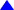Basic Statistics: Employees' Salary & Experience Variables

## Employees' Salary: Basic Statisticsb

Statistics

N Mean Median Mode Std. Deviation Variance Skewness Sum
Valid Missing
Statistic Statistic Statistic Statistic Statistic Statistic Statistic Statistic Std. Error Statistic
Salary of Employees 5 0 \$39,540.00 \$36,000.00 \$29,500(a) \$11,460.06 \$131,333,000.00 1.355 .913 \$197,700
a Multiple modes exist. The smallest value is shown

b. Note the following:

1. Earlier when we examined the histogram with the normal, it was concluded then that the underlying distribution is somewhat skewed to the right. The basic statistics or numerical summary measure validate that conclusion. Notice that the relationship among the three measures of the average or central tendency is such that the mean > median > mode, and the skewness coefficient has a value of 1.355 with a standard error of .913.

2. As noted in the footnote the inherent distribution has multiple modes, apparently due to the fact that there are two employees that earn between \$25,000 and \$35,000 in the first class interval and between \$35,000 and \$45,000 in the second class interval, thereby making it difficult to determine a unique modal value. SPSS/win resolves this situation by reporting the minimum value.

##### Employees' Experience (in years): Basic Statisticsb
Statistics

N Mean Median Mode Std. Deviation Variance Skewness Kurtosis Range Minimum Maximum
Valid Missing
Statistic Statistic Statistic Statistic Statistic Statistic Statistic Statistic Std. Error Statistic Std. Error Statistic Statistic Statistic
Years of Experience 5 0 7.40 7.00 2a 5.03 25.30 .806 .913 .449 2.000 13 2 15
a Multiple modes exist. The smallest value is shown
Years of Experience

Frequency Percent Valid Percent Cumulative Percent
Valid 2 1 20.0 20.0 20.0
4 1 20.0 20.0 40.0
7 1 20.0 20.0 60.0
9 1 20.0 20.0 80.0
15 1 20.0 20.0 100.0
Total 5 100.0 100.0
Total 5 100.0

Note the following:
1. Measures of Central Tendency: On average a typical employee has 7.40 years of experience using the arithmetic mean; however, the median suggests exactly 7 years while the modal value is not unique due to the existence of multiple modes in the data (as pointed out by the program in footnote 'a') because the values are non-repetitive. Hence, each value is potentially a candidate for the mode in this case.
2. Distribution: The skewness coefficient of .806 implies that the experience of the employees is somewhat skewed to the right. The Kurtosis coefficient of .449 indicates that the distribution is somewhat flat at the mode if a unique one were possible.
3. Frequency Table: In the table each employee belongs in a distinct category on the basis of experience; which is equivalent to 20% of the sample size of five employees.Top or Back to Descriptive Statistics or Exercises in the SPSS/win Primer or Home Page or Send me your Comments via E-mail